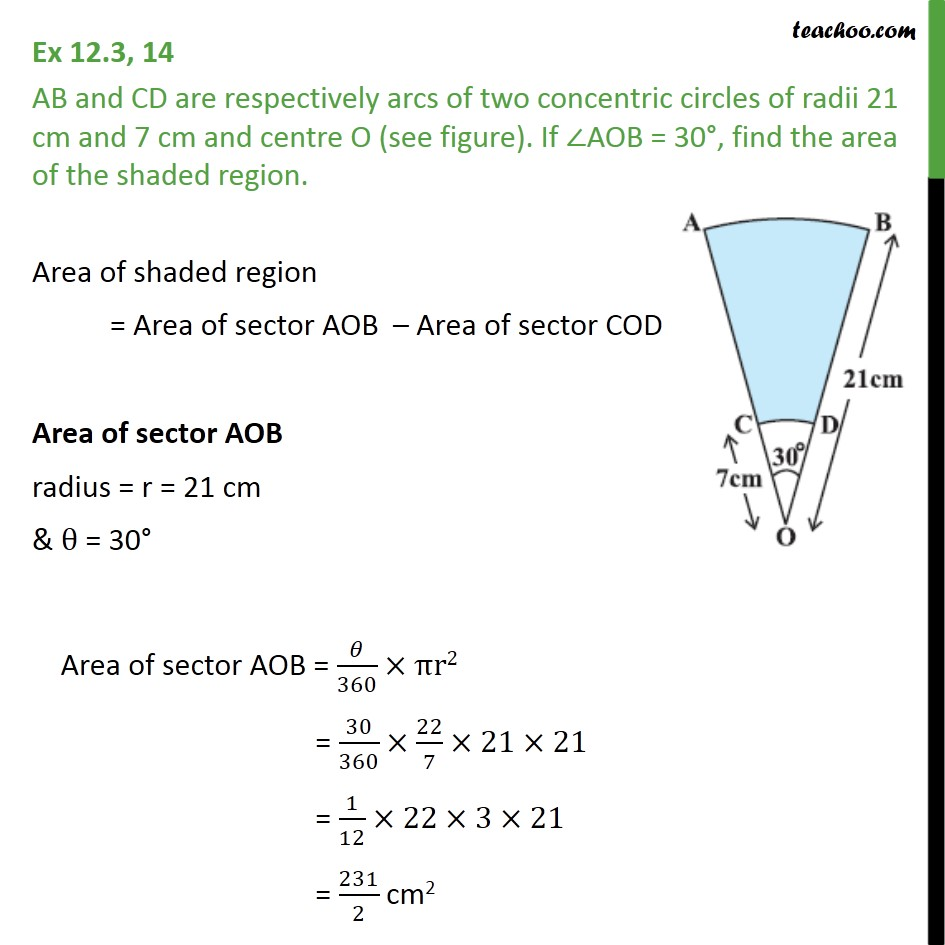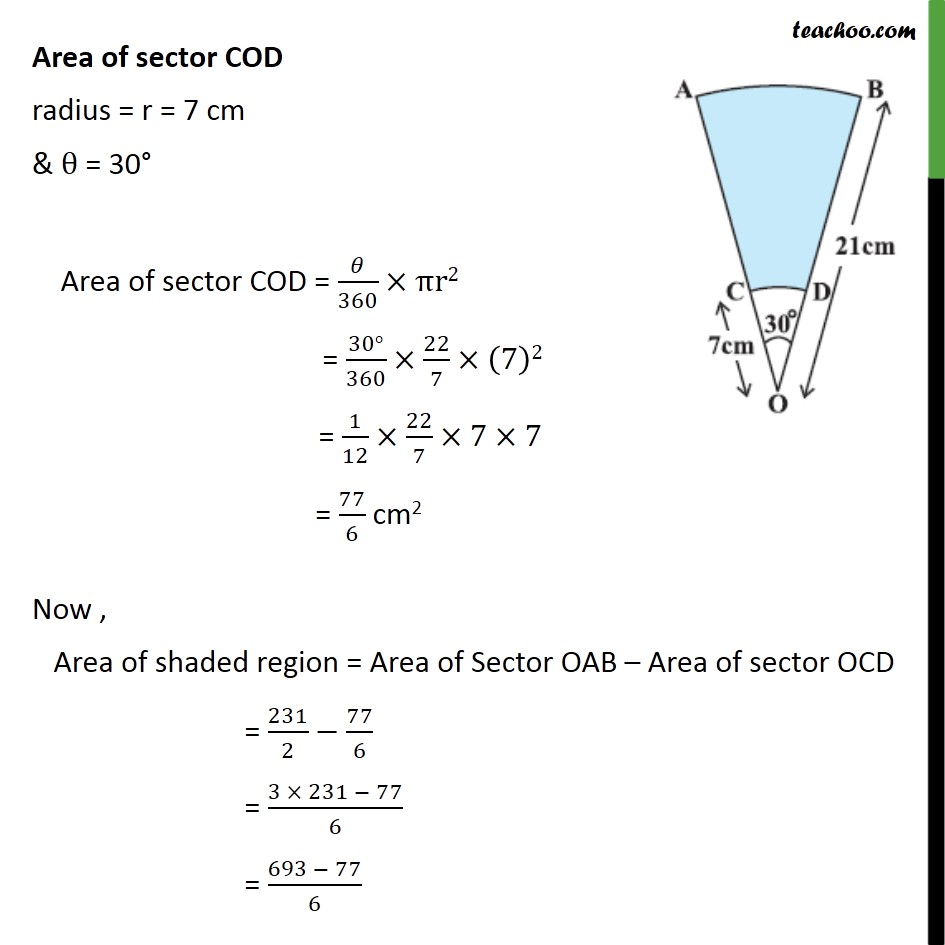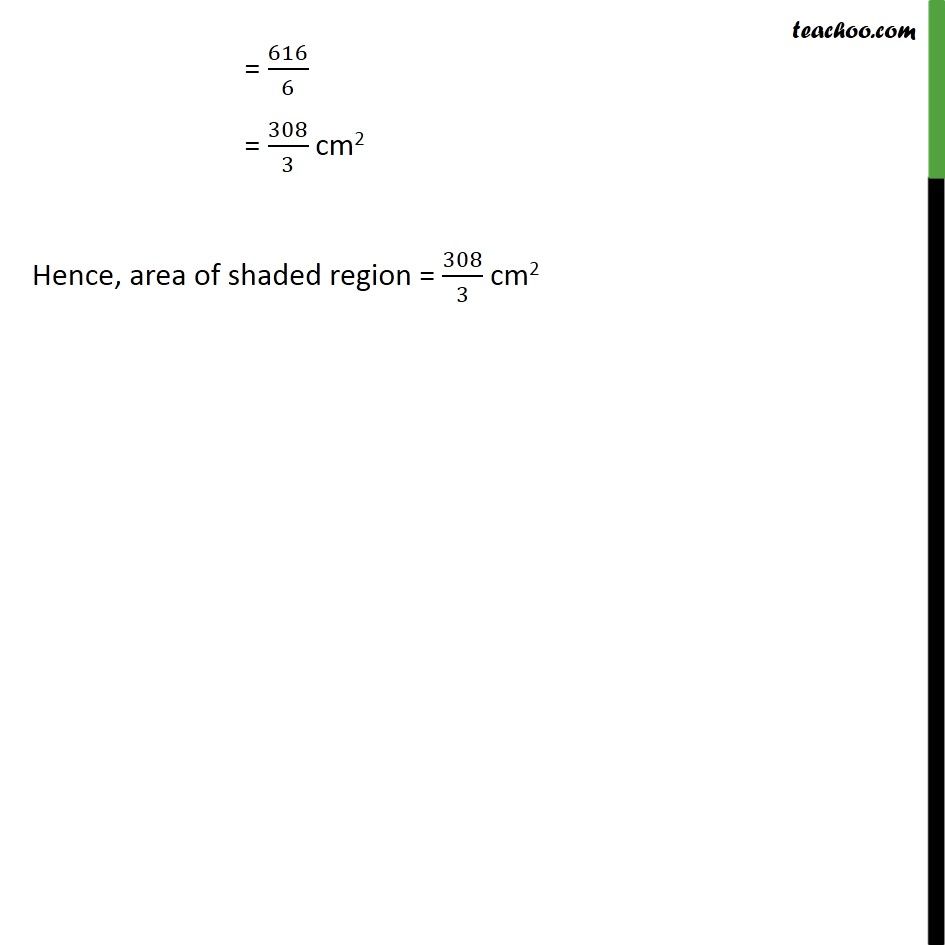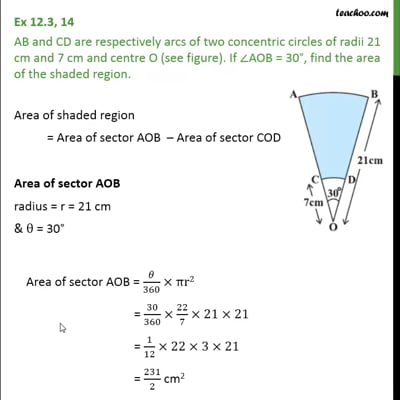Important Area Questions

Chapter 11 Class 10 Areas related to Circles
Serial order wiseThis video is only available for Teachoo black users

Learn in your speed, with individual attention - Teachoo Maths 1-on-1 Class

### Transcript

Question 14 AB and CD are respectively arcs of two concentric circles of radii 21 cm and 7 cm and centre O (see figure). If AOB = 30 , find the area of the shaded region. Area of shaded region = Area of sector AOB Area of sector COD Area of sector AOB radius = r = 21 cm & = 30 Area of sector AOB = /360 r2 = 30/360 22/7 21 21 = 1/12 22 3 21 = 231/2 cm2 Area of sector COD radius = r = 7 cm & = 30 Area of sector COD = /360 r2 = (30 )/360 22/7 (7)2 = 1/12 22/7 7 7 = 77/6 cm2 Now , Area of shaded region = Area of Sector OAB Area of sector OCD = 231/2 77/6 = (3 231 77)/6 = (693 77)/6 = 616/6 = 308/3 cm2 Hence, area of shaded region = 308/3 cm2